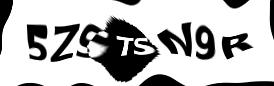As a reply to this challenge: Here is a small matcher for regular expressions, in Haskell (344 bytes):

``````p&(c:r)|c%h=p&z r|c%p=r:p&r|c%i=[]|1>0=p&r
z l=i&l!!0
b p q s=or[x?q\$s|x<-p:j&p]
[h,i,j]="()|"
(p@(f:r)?q)s|n%'?'=o?q#id|n%'*'=m#id|n%'+'=m#b x|f%h=b r(z r?q)s|f%i||f%j=q s where x|f%h=r|1>0=f:i:r;n:o=z x;m#c=c(o?q)s||b x m s;m c=c/=s&&(p?q)c
((f:r)?q)(d:v)|d%f=r?q\$v
(_?_)_=0>1
x%y=x==y
main=getLine>>=n.words
n(s:q)=mapM(print.b(s++[i])null)q``````

It reads a regular expression from the console (no character classes, no escape sequences or spaces), followed by several space-separated strings. Then, for each of the strings, it writes either `True` or `False`, depending on whether the string belongs to the language generated by the regular expression or not.

Who can descramble its inner workings? Who can write a shorter, but equivalent, Haskell program?

Contact and legal info | © 2016-07-31 siquod.org

## Discussion

No entries yetEnter the preceding text: